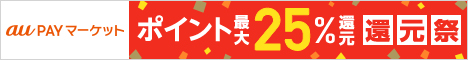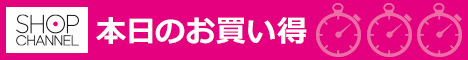# Java 运算符

## Java 运算符计算机的最基本用途之一就是执行数学运算，作为一门计算机语言，Java也提供了一套丰富的运算符来操纵变量。我们可以把运算符分成以下几组：
●算术运算符　　　●关系运算符
●位运算符　　　　●逻辑运算符
●赋值运算符　　　●其他运算符

+加法 - 相加运算符两侧的值A + B 等于 150
-减法 - 左操作数减去右操作数A – B 等于 -50
*乘法 - 相乘操作符两侧的值A * B等于5000
/除法 - 左操作数除以右操作数B / A等于2

++自增: 操作数的值增加1B++ 或 ++B 等于 101（区别详见下文）
--自减: 操作数的值减少1B-- 或 --B 等于 99（区别详见下文）

1 public class Test { public static void main(String[] args) { int a = 10; int b = 20; int c = 25; int d = 25; System.out.println(“a + b = ” + (a + b) ); System.out.println(“a – b = ” + (a – b) ); System.out.println(“a * b = ” + (a * b) ); System.out.println(“b / a = ” + (b / a) ); System.out.println(“b % a = ” + (b % a) ); System.out.println(“c % a = ” + (c % a) ); System.out.println(“a++ = ” + (a++) ); System.out.println(“a- – = ” + (a- -) ); // 查看 d++ 与 ++d 的不同 System.out.println(“d++ = ” + (d++) ); System.out.println(“++d = ” + (++d) ); } }

 a + b = 30 a – b = -10 a * b = 200 b / a = 2 b % a = 0 c % a = 5 a++ = 10 a– – = 11 d++ = 25 ++d = 27

1、自增（++）自减（- -）运算符是一种特殊的算术运算符，在算术运算符中需要两个操作数来进行运算，而自增自减运算符是一个操作数。

1 public class selfAddMinus{ public static void main(String[] args){ int a = 3;//定义一个变量 int b = ++a;//自增运算 int c = 3; int d = – -c;//自减运算 System.out.println(“进行自增运算后的值等于”+b); System.out.println(“进行自减运算后的值等于”+d); } }

 进行自增运算后的值等于4 进行自减运算后的值等于2

int b = ++a; 拆分运算过程为: a=a+1=4; b=a=4, 最后结果为b=4,a=4
int d = – -c; 拆分运算过程为: c=c-1=2; d=c=2, 最后结果为d=2,c=2
2、前缀自增自减法(++a,–a): 先进行自增或者自减运算，再进行表达式运算。
3、后缀自增自减法(a++,a–): 先进行表达式运算，再进行自增或者自减运算 实例：

1 public class selfAddMinus{ public static void main(String[] args){ int a = 5;//定义一个变量 int b = 5; int x = 2*++a; int y = 2*b++; System.out.println(“自增运算符前缀运算后a=”+a+“,x=”+x); System.out.println(“自增运算符后缀运算后b=”+b+“,y=”+y); } }

 自增运算符前缀运算后a=6，x=12 自增运算符后缀运算后b=6，y=10

==检查如果两个操作数的值是否相等，如果相等则条件为真。（A == B）为假
!=检查如果两个操作数的值是否相等，如果值不相等则条件为真。（A != B）为真
>检查左操作数的值是否大于右操作数的值，如果是那么条件为真。（A > B）为假
<检查左操作数的值是否小于右操作数的值，如果是那么条件为真。（A < B）为真
>=检查左操作数的值是否大于或等于右操作数的值，如果是那么条件为真。（A >= B）为假
<=检查左操作数的值是否小于或等于右操作数的值，如果是那么条件为真。（A <= B）为真

Test.java 文件代码：
1 public class Test { public static void main(String[] args) { int a = 10; int b = 20; System.out.println(“a == b = “ + (a == b) ); System.out.println(“a != b = “ + (a != b) ); System.out.println(“a > b = “ + (a > b) ); System.out.println(“a < b = " + (a < b) ); System.out.println(“b >= a = “ + (b >= a) ); System.out.println(“b <= a = " + (b <= a) ); } }

 a == b = false a != b = true a > b = false a < b = true b >= a = true b <= a = false

Java定义了位运算符，应用于整数类型(int)，长整型(long)，短整型(short)，字符型(char)，和字节型(byte)等类型。

 A = 0011 1100 B = 0000 1101 —————– A&B = 0000 1100 A | B = 0011 1101 A ^ B = 0011 0001 ~A= 1100 0011

|如果相对应位都是 0，则结果为 0，否则为 1（A | B）得到61，即 0011 1101
^如果相对应位值相同，则结果为0，否则为1（A ^ B）得到49，即 0011 0001

<<按位左移运算符。左操作数按位左移右操作数指定的位数A << 2得到240，即 1111 0000
>>按位右移运算符。左操作数按位右移右操作数指定的位数A >> 2得到15即 1111
>>>按位右移补零操作符。左操作数的值按右操作数指定的位数右移，移动得到的空位以零填充A>>>2得到15即0000 1111

Test.java 文件代码：
1 public class Test { public static void main(String[] args) { int a = 60; /* 60 = 0011 1100 */ int b = 13; /* 13 = 0000 1101 */ int c = 0; c = a & b; /* 12 = 0000 1100 */ System.out.println(“a & b = ” + c ); c = a | b; /* 61 = 0011 1101 */ System.out.println(“a | b = ” + c ); c = a ^ b; /* 49 = 0011 0001 */ System.out.println(“a ^ b = ” + c ); c = ~a; /*-61 = 1100 0011 */ System.out.println(“~a = ” + c ); c = a << 2; /* 240 = 1111 0000 */ System.out.println(“a << 2 = " + c ); c = a >> 2; /* 15 = 1111 */ System.out.println(“a >> 2 = ” + c ); c = a >>> 2; /* 15 = 0000 1111 */ System.out.println(“a >>> 2 = ” + c ); } }

 a & b = 12 a | b = 61 a ^ b = 49 ~a = -61 a << 2 = 240 a >> 2 = 15 a >>> 2 = 15

&&称为逻辑与运算符。当且仅当两个操作数都为真，条件才为真。（A && B）为假
| |称为逻辑或操作符。如果任何两个操作数任何一个为真，条件为真。（A | | B）为真

1 public class Test { public static void main(String[] args) { boolean a = true; boolean b = false; System.out.println(“a && b = ” + (a&&b)); System.out.println(“a || b = ” + (a||b) ); System.out.println(“!(a && b) = ” + !(a && b)); } }

 a && b = false a || b = true !(a && b) = true

1 public class Test { public static void main(String[] args) { int a = 5;//定义一个变量 boolean b = (a<4)&&(a++<10); System.out.println(“使用短路逻辑运算符的结果为”+b); System.out.println(“a的结果为”+a); } }

 使用短路逻辑运算符的结果为false a的结果为5

※解析： 该程序使用到了短路逻辑运算符(&&)，首先判断 a<4 的结果为 false，则 b 的结果必定是 false，所以不再执行第二个操作 a++<10 的判断，所以 a 的值为 5。

=简单的赋值运算符，将右操作数的值赋给左侧操作数C = A + B将把A + B得到的值赋给C
+ =加和赋值操作符，它把左操作数和右操作数相加赋值给左操作数C + = A等价于C = C + A
- =减和赋值操作符，它把左操作数和右操作数相减赋值给左操作数C - = A等价于C = C - A
* =乘和赋值操作符，它把左操作数和右操作数相乘赋值给左操作数C * = A等价于C = C * A
/ =除和赋值操作符，它把左操作数和右操作数相除赋值给左操作数C / = A，C 与 A 同类型时等价于 C = C / A
（％）=取模和赋值操作符，它把左操作数和右操作数取模后赋值给左操作数C％= A等价于C = C％A
<< =左移位赋值运算符C << = 2等价于C = C << 2
>> =右移位赋值运算符C >> = 2等价于C = C >> 2
＆=按位与赋值运算符C＆= 2等价于C = C＆2
^ =按位异或赋值操作符C ^ = 2等价于C = C ^ 2
| =按位或赋值操作符C | = 2等价于C = C | 2

 variable x = (expression) ? value if true : value if false

Test.java 文件代码：
1 public class Test { public static void main(String[] args){ int a , b; a = 10; // 如果 a 等于 1 成立，则设置 b 为 20，否则为 30 b = (a == 1) ? 20 : 30; System.out.println( “Value of b is : “ + b ); // 如果 a 等于 10 成立，则设置 b 为 20，否则为 30 b = (a == 10) ? 20 : 30; System.out.println( “Value of b is : “ + b ); } }

 Value of b is : 30 Value of b is : 20

instanceof 运算符

instanceof运算符使用格式如下：

 ( Object reference variable ) instanceof (class/interface type)

 String name = “James”; boolean result = name instanceof String; // 由于 name 是 String 类型，所以返回真

Test.java 文件代码：
1 class Vehicle {} public class Car extends Vehicle { public static void main(String[] args){ Vehicle a = new Car(); boolean result = a instanceof Car; System.out.println( result); } }

 true

Java运算符优先级

##### Java 循环结构